# Excel Date & Time

## Excel Date and Time Formating

Even though dates and time are actually stored as a regular number known as the date serial number, we can make use of extensive Excel date and time formatting options to display them just the way we want.

We can access some quick date and time formats from the Home tab in the Number group: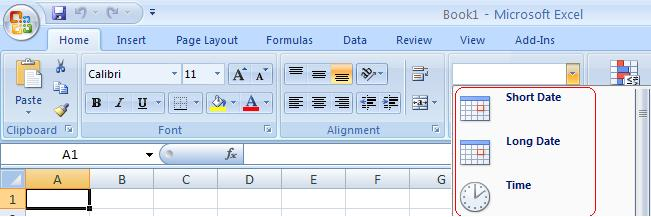## Custom Excel Date and Time Formating

We can also create our own custom date and time formats to suit our needs. Let's take a look.
1. Select the cell(s) containing the dates you want to format.
2. Press CTRL+1, or right-click > Format Cells to open the Format Cells dialog box.
3. On the Number tab select 'Date' in the Categories list. This brings up a list of default date formats you can select from in the 'Type' list. Likewise for the Time category.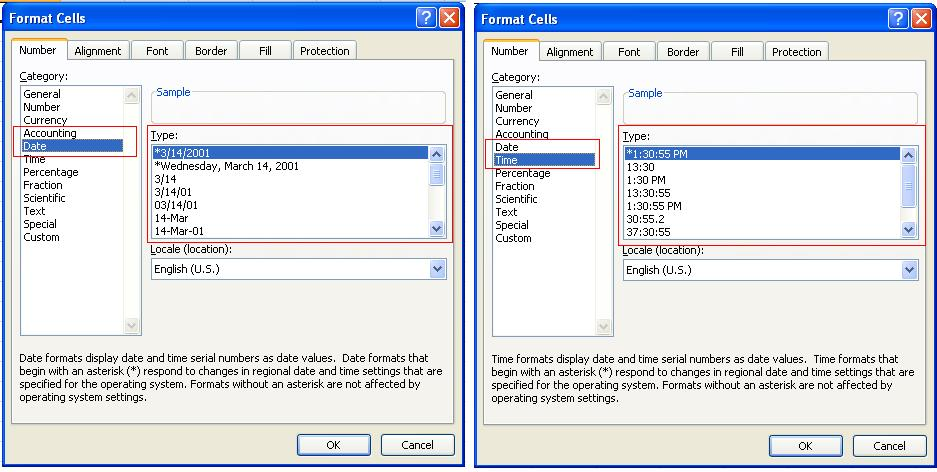We aren't limited to the defaults though. You can create your own Custom date or time formats in the 'Custom' category.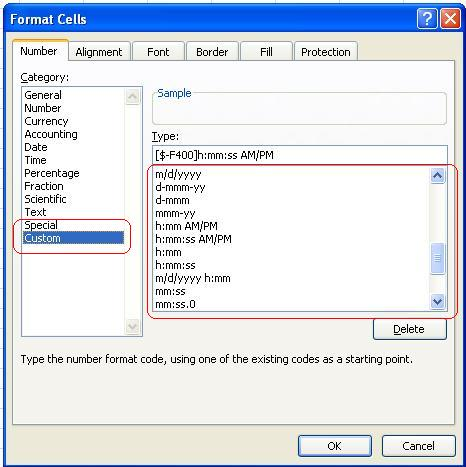## Understanding Excel Dates and Times

In Excel, we need to understand the way that Excel stores Date and Time, if you want to work with Excel Dates and Time.

Date and time are actually stored as simple numbers within Excel. It is only the Formatting of a spreadsheet's cells, that causes its contents to be displayed as a date, time, or date & time.

The following table shows how dates and times are coded within Excel:

For example :

1 = January 1, 1900
2 = January 2, 1900
42736 = January 1, 2017

Dates in Excel are stored as positive integers. Therefore, when entered into a worksheet, the integer values, 1, 2 and 42736 are displayed as follows (depending on the cell formatting):

With 'General'Formatting:

A
1 1
2 2
3 42736

With Date Format "mm/dd/yyyy":

A
1 01/01/1900
2 01/02/1900
3 01/01/2017

Time : Time in Excel are stored as decimals, between 0 and 1, which represent a proportion of the day.

For example:

0 = 00:00 hrs
0.25 = 06:00 hrs
0.5 = 12:00 noon

Therefore, when entered into a spreadsheet, the decimal values, 0, 0.25 and 0.5 are displayed as follows (depending on the cell formatting):

With 'General'Formatting:

A
1 0
2 0.25
3 0.5

With Time Format "hh:mm":

A
1 00:00
2 06:00
3 12:00

## Date & Time

Date & Time values in Excel are stored as decimals, comprised of:
• an integer (representing the date)
• a fraction between 0 and 1 (representing the time).
For example
1.5 = 12:00 hrs on January 1, 1900
2.25 = 06:00 hrs on January 2, 1900
42736.5 = 12:00 hrs on January 1, 2017

Therefore, when entered into a spreadsheet, the above values are displayed as follows (depending on the cell formatting):

=With 'General' Formatting:

A
1 1.5
2 2.25
3 42736.5

With Date & Time Format "mm/dd/yyyy hh:mm":

A
1 01/01/1900 12:00
2 01/02/1900 06:00
3 01/01/2017 12:00

Adding and Subtracting Dates and Times in Excel

Because Excel stores dates & times as numbers you can add, subtract and compare dates and times in Excel in the same way that you would add or subtract any other numbers.

Examples are provided below:

Example 1 - Calculate the Number of Days Between Two Dates

A B
1 08/11/2018 =A2-A1
2 08/29/2018

The Cell B1 contains the formula =A2-A1 to calculate the number of days between the dates 08/11/2018 & 08/29/2018 (stored in cells A1 and A2). This gives the result 18.

Example 2 - Calculate the Difference Between Two Times

A B
1 12:00 =A2-A1
2 18:45

Cell B1 uses the formula =A2-A1 to calculate the number of hours and minutes between the times 12:00 & 18:145 (stored in cells A1 and A2).This gives the result 06:45.

(Note that, in order to display this result, cell B1 should be formatted to have the time format "hh:mm").

Example 3 - Calculate the Difference Between Two Date and Time

A B
1 07/14/2018 20:00 =A2-A1
2 07/15/2018 07:00

The Cell B1 uses the formula =A2-A1 to calculate the number of hours and minutes between the two dates & times stored in cells A1 and A2. This gives the result 11:00.

(Note that, in order to display this result, cell B1 should be formatted to have the time format "hh:mm").

Example 4 - Add a Time to a Date and Time

A B
1 07/14/2017 20:00 =A1+A2
2 16:00

Cell B1 uses the formula =A1+A2 to calculate the date and time that is 20 hours after the date & time 07/14/2017 20:00. This gives the result 7/15/2018 12:00.

(Note that, in order to display this result, cell B1 should be formatted to have the date and time format "mm/dd/yyyyhh:mm").

## Formatting Dates and Times in Excel

The results of the above Excel date and time formulas may not have the required formatting when they are first entered into your spreadsheet. This may give the initial impression that the formula has not worked - however, this is not the case.

The cells can be made to show the correct result by formatting into a 'General', 'Date', 'Time', or 'Date & Time' format, depending on the required result type.

The easiest way to change the formatting of one or more Excel cells is to select the cell(s) to be formatted and then select the required formatting type from the drop-down menu in the ribbon. This is generally found in the Number group on the Home tab :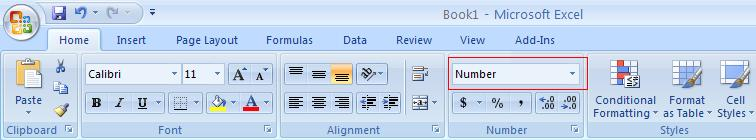If you don't see the formatting style that you require, select the option More Number Formats ... from the drop-down format menu. This opens up the 'Format Cells' dialog box, which provides several additional formats or allows you to define your own Custom format.

## How to Subtract Dates in Excel

As Excel dates are internally stored as simple integers, you can subtract dates in Excel, in the same way that you can subtract any other integers. I.e. to subtract dates in Excel:

1. Type the start date and the end date into your worksheet;
2. Subtract the start date from the end date;
3. Ensure the result is formatted as a number.

(The easiest way to do this is to select the cell and then select the 'General' format from the drop-down menu in the Home tab of the Excel ribbon).

The result from the subtraction formula is equal to the number of days between the two dates.

Excel Date Subtraction Examples

Column C of the following spreadsheet shows three examples of Excel date subtraction formulas. In each case, the date in column A is subtracted from the date in column B.

Formulas:

A B C
1 Start Date End Date Difference
2 6-May-2018 22-May-2018 =C1-B1
3 15-Aug-2018 11-Oct-2019 =C2-B2
4 1-Jan-2016 31-Dec-2019 =C3-B3

Results:

A B C
1 Start Date End Date Difference
2 6-May-2018 22-May-2018 16
3 15-Aug-2018 11-Oct-2019 422
4 1-Jan-2016 31-Dec-2019 1460

Note that, in the results above, the cells in columns A and B are formatted with a date format, but the cells in column C are formatted with the 'General' format.

## Changing Excel Cell Formatting

If the result of your date subtraction is displayed as a date, but you require a simple number, you will need to change the formatting of the results cell, to display a number.

The easiest way to do this is to select the General formatting option from the drop-down format menu of the Excel ribbon. This is generally located in the 'Number' group, on the Home tab of the ribbon :## How to Subtract Time in Excel

Subtraction of Time in Excel
As Time is stored as simple decimal values, you can subtract time in Excel in the same way that you can subtract any other numbers. Therefore, to subtract time in Excel:

1. Type the start time and the end time into your worksheet;
2. Sutract the start time from the end time;
3. Format the result as a time.

(The easiest way to format a worksheet cell to display a time is to select the cell and then select the 'Time' format from the drop-down menu in the Home tab of the Excel ribbon).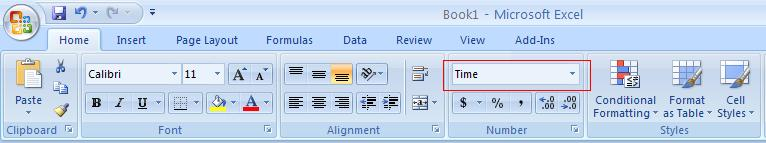Excel Time Subtraction Examples
Column C of the following spreadsheet shows three simple Excel time subtraction formulas.
In each case, the time in column A is subtracted from the time in column B.

Formulas:
A B C
1 Start Time End Time Time Difference
2 6:00 11:45 =C1-B1
3 12:45 20:20 =C2-B2
4 14:10:36 19:20:14 =C3-B3

Results:
A B C
1 Start Time End Time Time Difference
2 6:00 11:45 5:45
3 12:45 20:20 7:35
4 14:10:36 19:20:14 5:09:38

Note that, in the results above, cells C2 and C3 are formatted with the time format hh:mm and cells C4 use the time format hh:mm:ss.

## Formating Time in Excel

If you have a cell containing a positive decimal value, this can be diplayed as a time, by formatting the cell with a time format.
The easiest way to do this is to select the Time formatting from the drop-down format menu, which is generally located in the 'Number' group, on the Home tab of the Excel ribbon :

If you want to use a different time format, select More Number Formats ... from the drop-down format menu to open up the 'Format Cells' dialog box. Within this dialog box, you can select one of various Time formats or define your own Custom format.

To Learn Advanced Excel - Advanced Date & Time Functions Whatapp/Call 8422000829. Disount Code WEBSAB501

Home Chapter 1 Chapter 2 Chapter 3 Chapter 4 Chapter 5 Chapter 6 Chapter 7 Chapter 8 Chapter 10 Excel Shortcuts

Keep Learning...